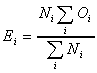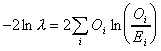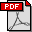# Log-likelihood and effect size calculator

To use this wizard, type in frequencies for one word and the corpus sizes and press the calculate button.
 Corpus 1 Corpus 2 Frequency of word Corpus size
Notes:
1. Please enter plain numbers without commas (or other non-numeric characters) as they will confuse the calculator!
2. The LL wizard shows a plus or minus symbol before the log-likelihood value to indicate overuse or underuse respectively in corpus 1 relative to corpus 2.
3. The log-likelihood value itself is always a positive number. However, my script compares relative frequencies between the two corpora in order to insert an indicator for '+' overuse and '-' underuse of corpus 1 relative to corpus 2.

## How to calculate log likelihood

Log likelihood is calculated by constructing a contingency table as follows:
 Corpus 1 Corpus 2 Total Frequency of word a b a+b Frequency of other words c-a d-b c+d-a-b Total c d c+d

Note that the value 'c' corresponds to the number of words in corpus one, and 'd' corresponds to the number of words in corpus two (N values). The values 'a' and 'b' are called the observed values (O), whereas we need to calculate the expected values (E) according to the following formula:In our case N1 = c, and N2 = d. So, for this word, E1 = c*(a+b) / (c+d) and E2 = d*(a+b) / (c+d). The calculation for the expected values takes account of the size of the two corpora, so we do not need to normalize the figures before applying the formula. We can then calculate the log-likelihood value according to this formula:This equates to calculating log-likelihood G2 as follows: G2 = 2*((a*ln (a/E1)) + (b*ln (b/E2)))

Note 1: (thanks to Stefan Th. Gries) The form of the log-likelihood calculation that I use comes from the Read and Cressie research cited in Rayson and Garside (2000) rather than the form derived in Dunning (1993).

Note 2: (thanks to Chris Brew) To form the log-likelihood, we calculate the sum over terms of the form x*ln(x/E). For strictly positive x it is easy to compute these terms, while if x is zero ln(x/E) will be negative infinity. However the limit of x*ln(x) as x goes to zero is still zero, so when summing we can just ignore cells where x = 0. Calculating ln(0) returns an error in, for example, MSExcel and the C-maths library.

The higher the G2 value, the more significant is the difference between two frequency scores. For these tables, a G2 of 3.8 or higher is significant at the level of p < 0.05 and a G2 of 6.6 or higher is significant at p < 0.01.

• 95th percentile; 5% level; p < 0.05; critical value = 3.84
• 99th percentile; 1% level; p < 0.01; critical value = 6.63
• 99.9th percentile; 0.1% level; p < 0.001; critical value = 10.83
• 99.99th percentile; 0.01% level; p < 0.0001; critical value = 15.13

## Effect size calculations

Alongside the Log Likelihood measure, the following effect size measures are implemented on this page:

• %DIFF - see Gabrielatos and Marchi (2012)
Costas has also provided an
FAQ with more details
• Bayes Factor (BIC) - see Wilson (2013)
You can interpret the approximate Bayes Factor as degrees of evidence against the null hypothesis as follows:
0-2: not worth more than a bare mention
2-6: positive evidence against H0
6-10: strong evidence against H0
> 10: very strong evidence against H0
For negative scores, the scale is read as "in favour of" instead of "against" (Wilson, personal communication).
• Effect Size for Log Likelihood (ELL) - see Johnston et al (2006)
ELL varies between 0 and 1 (inclusive). Johnston et al. say "interpretation is straightforward as the proportion of the maximum departure between the observed and expected proportions".
• Relative Risk - see links below
• Log Ratio - see Andrew Hardie's CASS blog for how to interpret this
Note that if either word has zero frequency then a small adjustment is automatically applied (0.5 observed frequency which is then normalised) to avoid division by zero errors.
• Odds Ratio - see links below

For a detailed comparison of the log-likelihood and chi-squared statistics, see
Rayson P., Berridge D. and Francis B. (2004). Extending the Cochran rule for the comparison of word frequencies between corpora. In Volume II of Purnelle G., Fairon C., Dister A. (eds.) Le poids des mots: Proceedings of the 7th International Conference on Statistical analysis of textual data (JADT 2004), Louvain-la-Neuve, Belgium, March 10-12, 2004, Presses universitaires de Louvain, pp. 926 - 936. ISBN 2-930344-50-4.The log-likelihood test can be used for corpus comparison. See
Rayson, P. and Garside, R. (2000). Comparing corpora using frequency profiling. In proceedings of the workshop on Comparing Corpora, held in conjunction with the 38th annual meeting of the Association for Computational Linguistics (ACL 2000). 1-8 October 2000, Hong Kong, pp. 1 - 6.For a more detailed review of various statistics, see:
Rayson, P. (2003). Matrix: A statistical method and software tool for linguistic analysis through corpus comparison. Ph.D. thesis, Lancaster University.And to read more about the use of log-likelihood with tag-level comparisons, see:
Rayson, P. (2008). From key words to key semantic domains. International Journal of Corpus Linguistics. 13:4 pp. 519-549. DOI: 10.1075/ijcl.13.4.06ray

The chi-square distribution calculator (Stat Trek) makes it easy to compute cumulative probabilities, based on the chi-square statistic.

The Institute of Phonetic Sciences in Amsterdam, have a similar calculator.

Also see Dunning, Ted. (1993). Accurate Methods for the Statistics of Surprise and Coincidence. Computational Linguistics, Volume 19, number 1, pp. 61-74. (pdf)

Andrew Hardie has created a significance test system which calculates Chi-squared, log-likelihood and the Fisher Exact Test for contingency tables using R.

There is an increasing movement in corpus linguistics and other fields (e.g. Psychology) to move away from null hypothesis testing and p-values, and to calculate effect size measures as well as significance values. For a discussion of these measures and why we need them, see the following resources, presentations and publications:

There are a number of other papers related to the use of significance testing, keyness statistics and corpus comparison, e.g. Kilgarriff (2005), Paquot and Bestgen (2009), Baron et al. (2009), Wilson (2013) and Lijffijt et al.